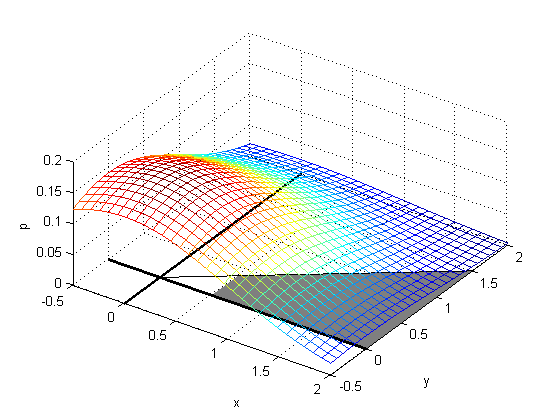#Boost C++ Libraries

...one of the most highly regarded and expertly designed C++ library projects in the world.

## Owen's T function

##### Synopsis
```#include <boost/math/special_functions/owens_t.hpp>
```
```namespace boost{ namespace math{

template <class T>
calculated-result-type owens_t(T h, T a);

template <class T, class Policy>
calculated-result-type owens_t(T h, T a, const Policy&);

}} // namespaces
```
##### Description

Returns the Owens_t function of h and a.

The final Policy argument is optional and can be used to control the behaviour of the function: how it handles errors, what level of precision to use etc. Refer to the policy documentation for more details.The function `owens_t(h, a)` gives the probability of the event (X > h and 0 < Y < a * X), where X and Y are independent standard normal random variables.

For h and a > 0, T(h,a), gives the volume of an uncorrelated bivariate normal distribution with zero means and unit variances over the area between y = ax and y = 0 and to the right of x = h.

That is the area shaded in the figure below (Owens 1956).and is also illustrated by a 3D plot.This function is used in the computation of the Skew Normal Distribution. It is also used in the computation of bivariate and multivariate normal distribution probabilities. The return type of this function is computed using the result type calculation rules: the result is of type `double` when T is an integer type, and type T otherwise.

Owen's original paper (page 1077) provides some additional corner cases.

T(h, 0) = 0

T(0, a) = ½π arctan(a)

T(h, 1) = ½ G(h) [1 - G(h)]

T(h, ∞) = G(|h|)

where G(h) is the univariate normal with zero mean and unit variance integral from -∞ to h.

##### Accuracy

Over the built-in types and range tested, errors are less than 10 * std::numeric_limits<RealType>::epsilon().

Table 8.86. Error rates for owens_t

GNU C++ version 7.1.0
linux
double

GNU C++ version 7.1.0
linux
long double

Sun compiler version 0x5150
Sun Solaris
long double

Microsoft Visual C++ version 14.1
Win32
double

Owens T (medium small values)

Max = 0ε (Mean = 0ε)

Max = 3.34ε (Mean = 0.944ε)

Max = 3.34ε (Mean = 0.911ε)

Max = 4.37ε (Mean = 0.98ε)

Owens T (large and diverse values)

Max = 0ε (Mean = 0ε)

Max = 49ε (Mean = 2.16ε)

Max = 24.5ε (Mean = 1.39ε)

Max = 3.78ε (Mean = 0.621ε)

##### Testing

Test data was generated by Patefield and Tandy algorithms T1 and T4, and also the suggested reference routine T7.

• T1 was rejected if the result was too small compared to `atan(a)` (ie cancellation),
• T4 was rejected if there was no convergence,
• Both were rejected if they didn't agree.

Over the built-in types and range tested, errors are less than 10 std::numeric_limits<RealType>::epsilon().

However, that there was a whole domain (large h, small a) where it was not possible to generate any reliable test values (all the methods got rejected for one reason or another).

There are also two sets of sanity tests: spot values are computed using Wolfram Mathematica and The R Project for Statistical Computing.

##### Implementation

The function was proposed and evaluated by Donald. B. Owen, Tables for computing bivariate normal probabilities, Ann. Math. Statist., 27, 1075-1090 (1956).

The algorithms of Patefield, M. and Tandy, D. "Fast and accurate Calculation of Owen's T-Function", Journal of Statistical Software, 5 (5), 1 - 25 (2000) are adapted for C++ with arbitrary RealType.

The Patefield-Tandy algorithm provides six methods of evaluation (T1 to T6); the best method is selected according to the values of a and h. See the original paper and the source in owens_t.hpp for details.

The Patefield-Tandy algorithm is accurate to approximately 20 decimal places, so for types with greater precision we use:

• A modified version of T1 which folds the calculation of atan(h) into the T1 series (to avoid subtracting two values similar in magnitude), and then accelerates the resulting alternating series using method 1 from H. Cohen, F. Rodriguez Villegas, D. Zagier, "Convergence acceleration of alternating series", Bonn, (1991). The result is valid everywhere, but doesn't always converge, or may become too divergent in the first few terms to sum accurately. This is used for ah < 1.
• A modified version of T2 which is accelerated in the same manner as T1. This is used for h > 1.
• A version of T4 only when both T1 and T2 have failed to produce an accurate answer.
• Fallback to the Patefiled Tandy algorithm when all the above methods fail: this happens not at all for our test data at 100 decimal digits precision. However, there is a difficult area when a is very close to 1 and the precision increases which may cause this to happen in very exceptional circumstances.

Using the above algorithm and a 100-decimal digit type, results accurate to 80 decimal places were obtained in the difficult area where a is close to 1, and greater than 95 decimal places elsewhere.

 Copyright © 2006-2021 Nikhar Agrawal, Anton Bikineev, Matthew Borland, Paul A. Bristow, Marco Guazzone, Christopher Kormanyos, Hubert Holin, Bruno Lalande, John Maddock, Evan Miller, Jeremy Murphy, Matthew Pulver, Johan Råde, Gautam Sewani, Benjamin Sobotta, Nicholas Thompson, Thijs van den Berg, Daryle Walker and Xiaogang Zhang Distributed under the Boost Software License, Version 1.0. (See accompanying file LICENSE_1_0.txt or copy at http://www.boost.org/LICENSE_1_0.txt)